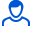Content: d8-37.zip (89.59 KB)

Positive responses: 0
Negative responses: 0

Sold: 0
Refunds: 0Loyalty discount! If the total amount of your purchases from the seller more than:

 \$1 the discount is 1% \$3 the discount is 2% \$5 the discount is 3% \$15 the discount is 5% \$30 the discount is 7% \$50 the discount is 10% \$250 the discount is 15%

The finished solution of the D variant 8 of 37 Reshebnik on theoretical mechanics SM Targ 1983 - (. Razg termehu Targ). D8 conditions of the problem (p. 56-59 in the training manual Targ SM 1983.): The mechanical system consists of bodies 1, 2, ..., 5, having a weight of P1, P2, ..., the P5, svjazanyh yarns wound on stepped blocks 1 and 2 (Fig. D8.0, D8.9, Table. D8). A dash in the table columns, where the weights are set, indicates that the corresponding body not included in (the figure does not depict), a zero in the columns of the table means that the body is considered weightless but in part (in the figure should be shown). For wheels, designated No. 4, P4 - their total weight (a weight of the platform carriage is not considered). The radii of the stepped blocks 1 and 2 are, respectively: R1 = R, r1 = 0,8R; R2 = R, r2 = 0,4 R. To calculate the moments of inertia of both blocks, wheels and rollers considered homogeneous cylinder of radius R. In the system of forces other than gravity force are applied to the body F. 4 or 5 (if the body 5 is not included in , force is applied at the point in the trolley), and a pair of forces with moments M1 and M2 are applied to blocks (for M <0 the direction opposite to the time shown in the figure). Thread on the plot indicated in the table under "Spring", incorporated with spring stiffness coefficient s (for example, if the column should AB, AB is the portion of the spring, if the AD AD spring, etc.); at the initial time the spring is not deformed.
Create for the Lagrange equations of the system and to determine the frequency of them and the period of oscillation, made system bodies while moving.
After payment you will receive a personalized link to the unique solution of the problem D8 Option 37 on the theoretical mechanics of a single SM Reshebnik Targ 1983.
The decision coincides with the solution of the problem of D6 Targ 1988.
The decision is made on the job methodical instructions and control tasks termehu for part-time students of thermal power, mining, metallurgy, electro-instrument-making, automation and technological specialties universities.
The decision is decorated in a word / pdf format / less hand-written in jpg or gif format verify if necessary.